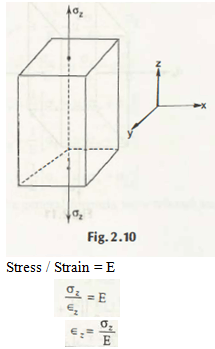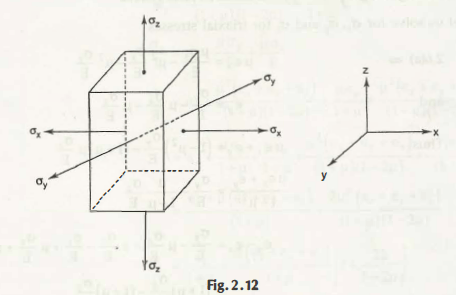What do you mean by Poisson’s Ratio?

The exact ratio of Lateral Strain to the Longitudinal Strain is known as Poisson’s ratio. It is denoted by μ. So, you can write μ = The lateral strain / The longitudinal strain.

Uni – axial stress system

To understand this in a proper way, you just need to go through the following diagramYou can see here a bar is lengthened by an axial tension where a reduction will be taken place in transverse dimensions. The expert Poinssion concluded and showed that in case of the unit deformation ratio or you can say the strain in these sides or directions is constant for the exact stresses as there is proportional limit.

And now you can say that Stress/ Strain  = E

Now, if the direction of Z is getting increased with its dimension, then the dimension in the direction x and y will be decreased.

So, clearly the law can easily be written as –

Lateral Strain/longitudinal strain

= – εx / εz = -εy / εz

If you want to know that what is E here, then it is the property of a material. And μ is required to find out properly as it is also a property of the material.

µ = – εxz

εx = – µ εz = – µ x σz / E

εy= – µ . σz / E

εz =  σz / E.

Here is the strain in x, y and z direction while you have the perfect loading on z direction.

Biaxial Stress System

In bi-axial stress is described in two different directions suppose σx and σz. In this z direction dimension increases and σx influences the length in X direction.

εz =  σz / E. – µ . σx / E

εx =  σx / E. – µ . σz / E

εy =  σx/ E. – µ . σz / E

This is explained properly because of the loading in x and z direction. The strain can be seen in x, y and z direction.

Tri-axial Stress System

The strain can be determined as the summation Poisson’s effect and direct effect.+ Direct effect

-Poisson’s effect

εx =  σx / E. – µ. σy / E . – µ σz / E

εy =  σy / E. – µ . σx / E. – µ σz / E

εz =  σz/ E. – µ .  σx / E – µ σz / E

εx = 1/ E [ σx – µ (σy + σz)]

εy  =1/ E [ σy – µ (σx + σz)]

εz =1/ E [ σz – µ (σz + σy)]

These are the equations of the Triaxial Stress system. All the stresses present in these equations are tensiles. In case of some particular conditions when the stresses are compressive, then it can be changed adequately in case of signs.

ax and ay.for biaxial stresses

There are different conditions in which the stresses are bi-axials. Thus, to acquire the perfect solution, you can easily get

σx = ( εx + µεy) E / 1-µ2

for biaxial stresses

σy = (εy + µεx) E / 1-µ2

Links of Next Mechanical Engineering Topics:-### Customer Reviews

My Homework Help
Rated 5.0 out of 5 based on 510 customer reviews at